# 羡慕实时数据看板？来看看Python的交互数据分析可视化工具！ ⛵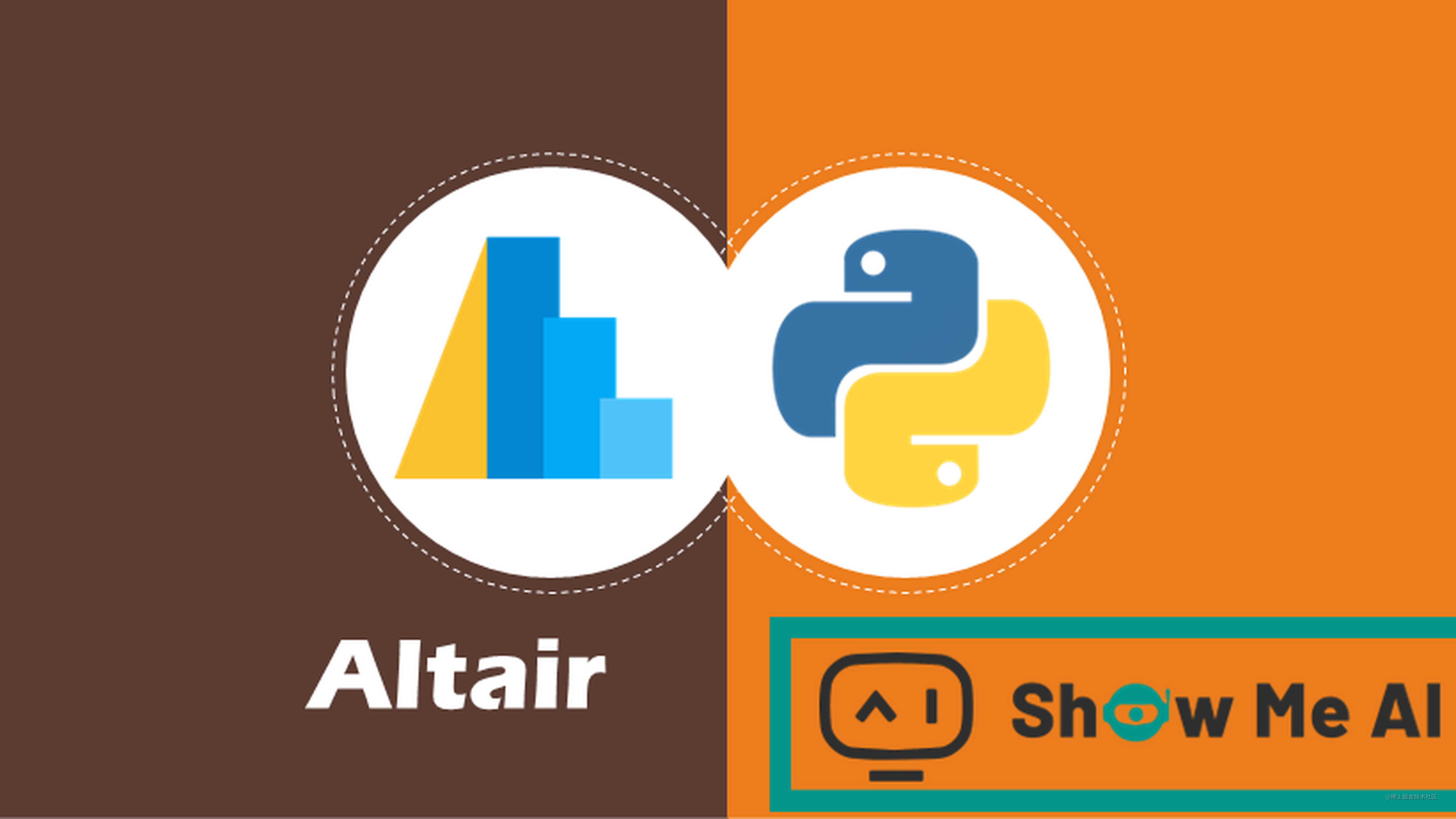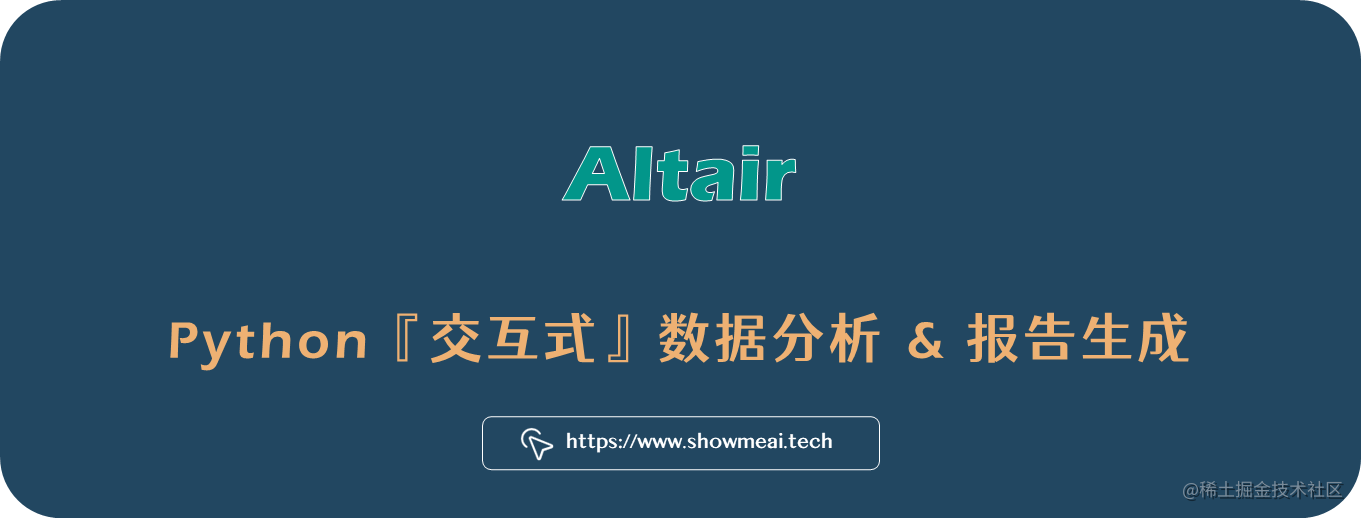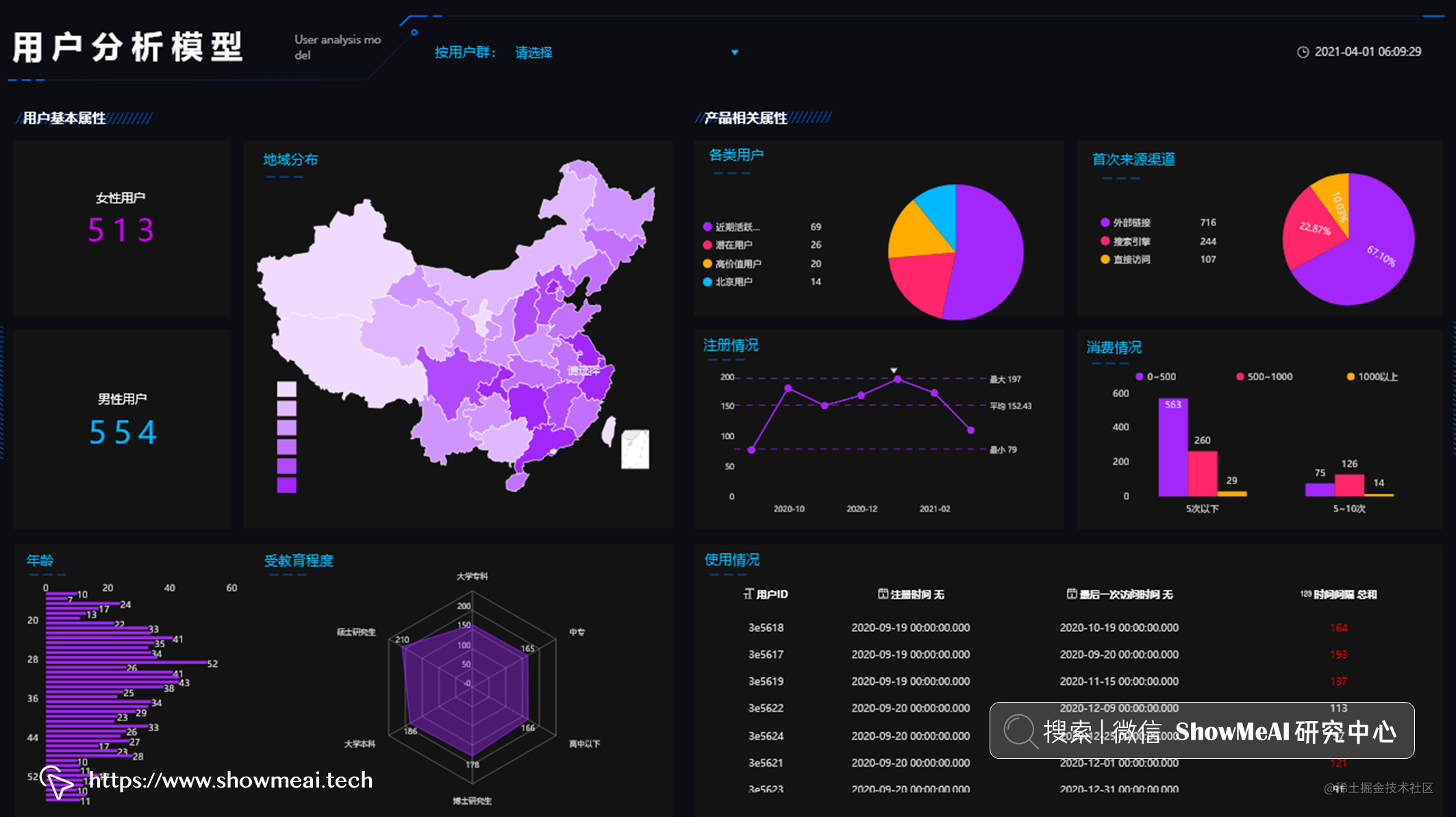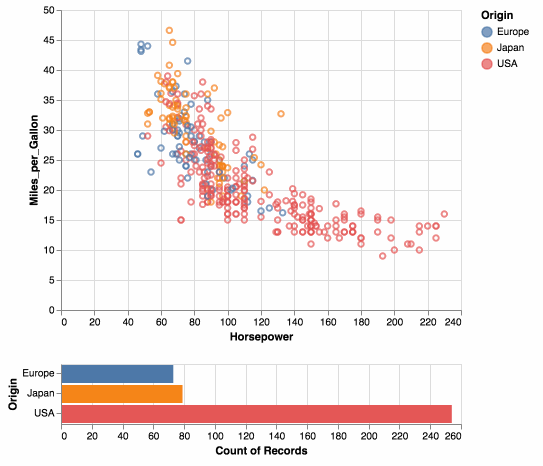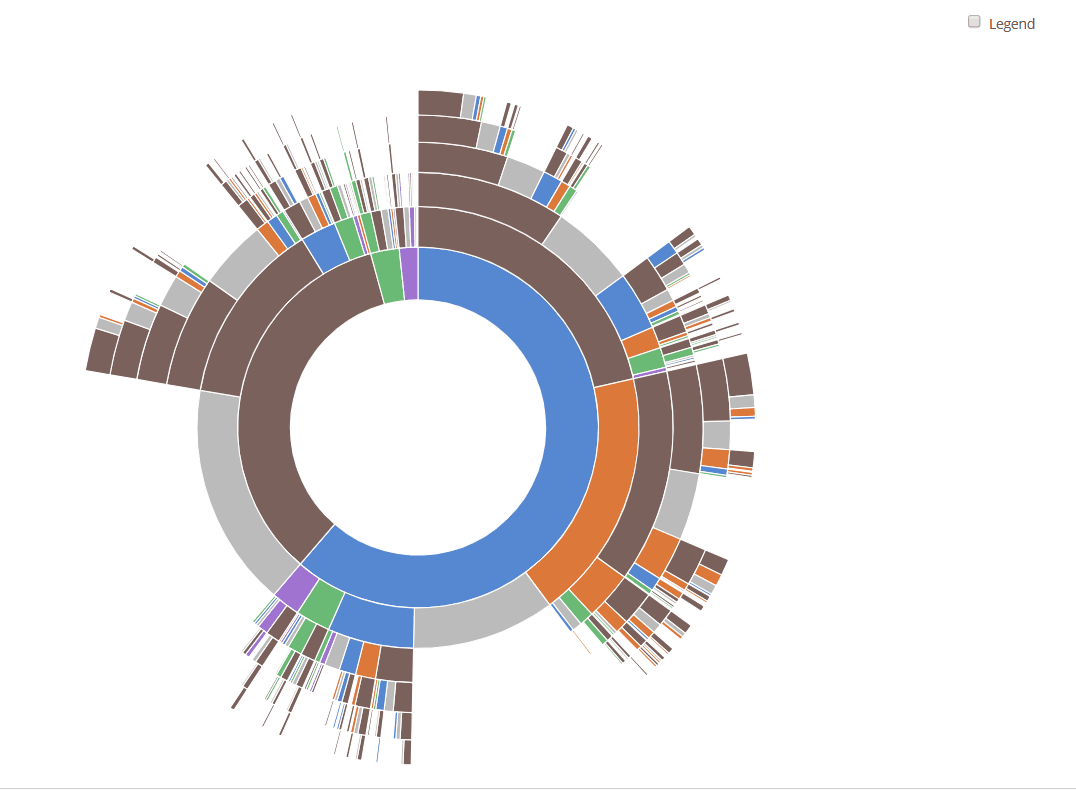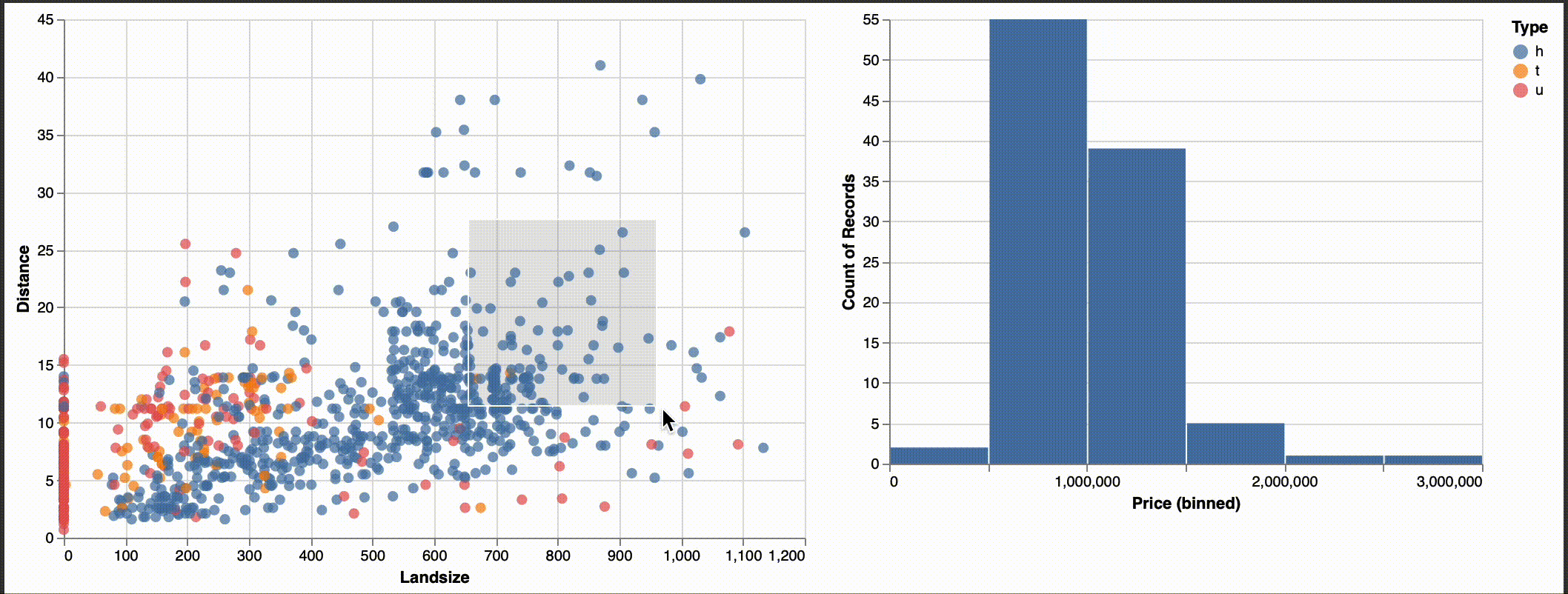# 💡 数据分析实现模板

``````# 导入工具库
import altair as alt
import pandas as pd

# 忽律数据规模限制
alt.data_transformers.enable('default', max_rows=None)

# 构建chart函数，它读取数据和字段名称，返回一个交互式图表结果
def chart(dataset, column_name, target_var):
w = 500
single = alt.selection_single()

# 灰度图与柱状图

# 如果是类别型字段，我们不用分桶
if (column_name in dataset.select_dtypes(include='object').columns.to_list()):
a = alt.Chart(dataset).mark_bar().encode(
alt.X(column_name + ':N', bin=False),
alt.Y('count()'),
color = alt.condition(single, alt.value('#4c78a8'), alt.value('lightgray')),
tooltip=['count()', alt.Tooltip(column_name, bin=False)]

# 如果是数值型字段，我们先分桶
else:
a = alt.Chart(dataset).mark_bar().encode(
alt.X(column_name + ':Q', bin=True),
alt.Y('count()'),
color = alt.condition(single, alt.value('#4c78a8'), alt.value('lightgray')),
tooltip=['count()', alt.Tooltip(column_name, bin=True)]

# 对于类别型字段，我们构建它和目标字段的一个箱线图表；对于数值型字段，我们构建它们和目标字段的散点分布图
try:
if (column_name in dataset.select_dtypes(include='object').columns.to_list()):
b = alt.Chart(dataset).mark_boxplot().encode( #, title="Boxplot of " + column_name
alt.X(column_name + ':N'),
alt.Y(target_var),
color = alt.condition(single, alt.value('#4c78a8'), alt.value('lightgray')),
tooltip=[target_var]
else:
b = alt.Chart(dataset).mark_point().encode(
alt.X(column_name + ':Q'),
alt.Y(target_var),
color = alt.condition(single, alt.value('#4c78a8'), alt.value('lightgray')),
tooltip=[target_var]
except:
pass

return(a | b)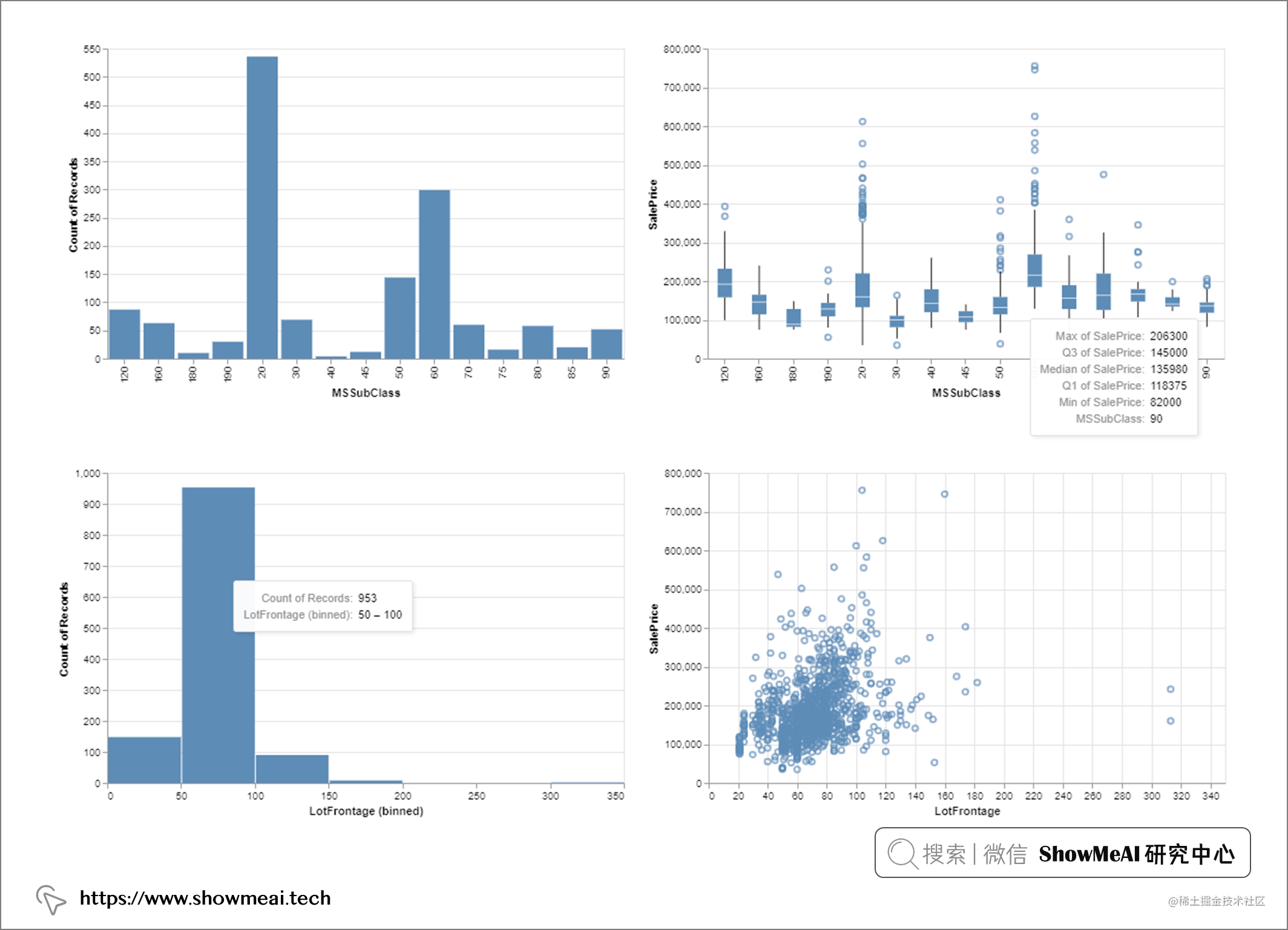# 💡 数据分析&交互文档报告

``````# 把所有的altair图表添加到一个列表里
myl = []
for col in dataset.columns:
try:
myl.append(chart(dataset, col, target_var))
except:
pass
else:
pass

# 编译所有的图表到1个html文件中
a = myl
for i in range(1,len(myl)):
a = a & myl[i]
a.properties(
title = 'Feature Histograms & Boxplots'
).configure_axis(labelFontSize=15, titleFontSize=25)
a.save('figures/Feature_Visuals.html')

# 检查是否所有的字段都可以被可视化
print('Features accounted for:', len(myl), 'out of', len(dataset.T))

# 参考资料